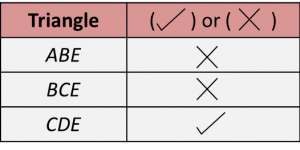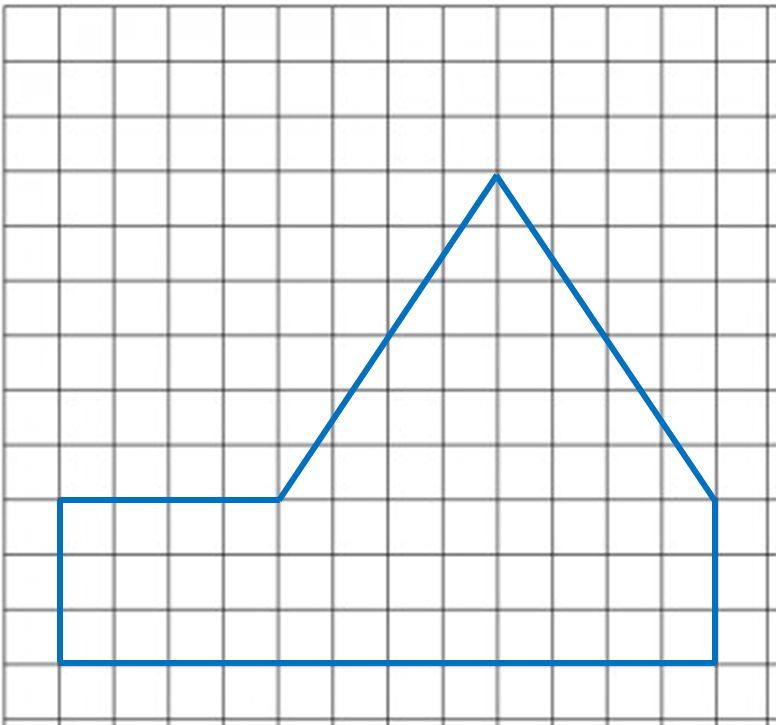# PT3 Mathematics 2016, Question 6

  
     
Question 6 (a):
  
(i) Fill in the blanks with the correct answer.(ii) Diagram 6.1 shows a set of triangles.On the answer space, mark (\/) for the right-angled triangles and mark (x) for the not right-angled triangles.
  
  Answer:Solution:
   (i)
     $\begin{array}{l}{k}^{2}={\overline{)m}}^{2}+{\overline{)n}}^{2}\\ \\ x=\sqrt{{\overline{)5}}^{2}-{\overline{)4}}^{2}}\end{array}$
  
(ii)

  In ∆ABE,
  AE2 = AB2 + BE2
  
= 8 2 + 172
  
= 353
  ≠ 192
  
Therefore, ∆ABE is not a right-angled triangle.
  
  In ∆BCE,
  BE2 = BC2 + CE2
  
= 9 2 + 122
  
= 152
  
≠ 172
  
Therefore, ∆BCE is not a right-angled triangle.
  
  In ∆CDE,
  CD2 = CE2 + DE2
  
= 12 2 + 52
  
= 169
  = 132
  
Therefore, ∆CDE is a right-angled triangle.Question 6 (b):
   
Diagram 6.1 shows a polygon.(a) If the polygon is redrawn using the scale 1 : 500, calculate the length of side drawn for the side 15 m.
   (b) On the square grids in the answer space, redraw the polygon using the scale 1 : 500. The grid has equal squares with sides of 1 cm.
   
  Solution:
(a)
          
   
   (b)Question 6 (c):
  
Diagram 6.3 shows a solid cuboid and a solid cube. The base of the cuboid is a square with sides 9 cm.The total surface of area of the cuboid and the cube are equal.
  Calculate the length of the side of the cube.
  
  Solution: